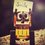# Range of a function

Need help in this

Find the range of $\sqrt{f(x)-g(x)} - \sqrt{f(x)+g(x)}$

Such that range of $f(x)$ is $(a,b)$ for all $x \in R$

And range of $g(x)$ is $(c,d)$ $x \in R$

Where $a,c \in R^{-} \space and \space b,d \in R^{+}$

$f(x)$ and $g(x)$ are continous and both are increasing functions.Note by Kushal Patankar
5 years, 11 months ago

This discussion board is a place to discuss our Daily Challenges and the math and science related to those challenges. Explanations are more than just a solution — they should explain the steps and thinking strategies that you used to obtain the solution. Comments should further the discussion of math and science.

When posting on Brilliant:

• Use the emojis to react to an explanation, whether you're congratulating a job well done , or just really confused .
• Ask specific questions about the challenge or the steps in somebody's explanation. Well-posed questions can add a lot to the discussion, but posting "I don't understand!" doesn't help anyone.
• Try to contribute something new to the discussion, whether it is an extension, generalization or other idea related to the challenge.
• Stay on topic — we're all here to learn more about math and science, not to hear about your favorite get-rich-quick scheme or current world events.

MarkdownAppears as
*italics* or _italics_ italics
**bold** or __bold__ bold
- bulleted- list
• bulleted
• list
1. numbered2. list
1. numbered
2. list
Note: you must add a full line of space before and after lists for them to show up correctly
paragraph 1paragraph 2

paragraph 1

paragraph 2

[example link](https://brilliant.org)example link
> This is a quote
This is a quote
    # I indented these lines
# 4 spaces, and now they show
# up as a code block.

print "hello world"
# I indented these lines
# 4 spaces, and now they show
# up as a code block.

print "hello world"
MathAppears as
Remember to wrap math in $$ ... $$ or $ ... $ to ensure proper formatting.
2 \times 3 $2 \times 3$
2^{34} $2^{34}$
a_{i-1} $a_{i-1}$
\frac{2}{3} $\frac{2}{3}$
\sqrt{2} $\sqrt{2}$
\sum_{i=1}^3 $\sum_{i=1}^3$
\sin \theta $\sin \theta$
\boxed{123} $\boxed{123}$

## Comments

Sort by:

Top Newest

Don't you think your question is too vague??

- 5 years, 11 months ago

Log in to reply

Yep, i got that now. It was just crazy curiosity

- 5 years, 11 months ago

Log in to reply

This question is too vague. We cannot proceed further without some information about the functions given . please try to expkain further.

- 5 years, 11 months ago

Log in to reply

What would be further necessary information.

- 5 years, 11 months ago

Log in to reply

We do not know anything about the fuctions. First of all we dont know whether they are continuous. Secondly, we dont know what values they take. For example assume f() takes some value in its range for some x, we dont know the respective value of g() for that x.. It may even be possible for the given expression to become undefined. But we cant say anything since we dont know f and g. If f and g are given, then it is possible.. Otherwise, i dont think we can do it.

We may be able to devise a set method or tactics to solve problems like this when f and g are given. We may be able to find a weak range.. Nothing more.

- 5 years, 11 months ago

Log in to reply

I added some information and hope thats enough

- 5 years, 11 months ago

Log in to reply

Nope.. Even now, it is ambiguous. Consider something like f(x)=x and g(x)=x+1 for x between some negative number and some positive number. Here, there is no element in range as given expression is undefined everywhere.

- 5 years, 11 months ago

Log in to reply

Does it matter whether $f(x)$ and $g(x)$ are continous?

- 5 years, 11 months ago

Log in to reply

×

Problem Loading...

Note Loading...

Set Loading...